American Journal of Theoretical and Applied Statistics
Volume 5, Issue 2, March 2016, Pages: 64-69

Comparison of Tests of Indirect Effect in Single Mediation Analysis

Chike Henry Nwankwo1, Amechi Henry Igweze2

1Department of Statistics, Nnamdi Azikiwe University, Awka, Nigeria

2Department of Mathematics and Statistics, Delta State Polytechnic, Ogwashi-Uku, Nigeria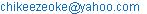(C. H. Nwankwo)(A. H. Igweze)

Chike Henry Nwankwo, Amechi Henry Igweze. Comparison of Tests of Indirect Effect in Single Mediation Analysis. American Journal of Theoretical and Applied Statistics. Vol. 5, No. 2, 2016, pp. 64-69. doi: 10.11648/j.ajtas.20160502.14

Received: January 16, 2016; Accepted: February 4, 2016; Published: March 18, 2016

Abstract: This study compares various methods of mediations analysis. Firstly, it compares the two methods of calculating indirect effect which are product of coefficient and difference of coefficients respectively. Secondly, the study compares the three methods of testing the significance of indirect effect vis avis Sobel’s test, Aroian test and Goodman’s test. The differences in these three tests are due to variations in the methods of standard error computation. The findings are discussed. The results show that both methods of product of coefficients and difference of coefficients give approximately the same result. However the product of coefficient gave a slightly higher result. The comparison of test of indirect effect for mediator shows that the tests gave the same result for Sobel’s, Aroian and Goodman test. The study recommended further studies to seek methods of ascertaining the direction of relationship of indirect effect, other than those of the regression models, further studies may be carried out to determine the effect of multicolinearity on mediation results.

Keywords: Mediation, Sobels Test, Aroian Test, Goodman Test, Indirect Effect

Contents

1. Introduction

A primary purpose of research is to identify the relationship between two variables. Often, theory suggests that a third variable may improve understanding of the nature of the relationship between the two primary variables. The third variable considered a mediator, is hypothesized to be linked in a causal chain between the independent and dependent variables. In other words, the independent variable causes the mediator and the mediator causes the dependent variable. The search for intermediate causal variables is called mediation analysis.

Fairchild & MacKinnon  noted that a mediation model is one that seeks to identify and explain the mechanism or process that underlies an observed relationship between an independent variable and a dependent variable via the inclusion of a third explanatory variable, known as a mediator variable.

The term statistical mediation or simply mediation, refers to a causal chain in which it is assumed that the effect of one or more independent variables is transmitted to one or more dependent variables through third variables. In the simplest case, the term mediation is used to indicate that the effect of an independent variable X is transmitted to a dependent variable Y through a third mediator variable Z. Therefore, statistical mediation refers to a causal sequence such as X → Z → Y. A mediator variable is very useful to help understand the mechanism through which a cause (independent variable) produces an effect (dependent variable) .

According to MacKinnon , there are three major approaches to statistical mediation analysis: (a) causal steps, (b) difference in coefficients, and (c) product of coefficients All of these methods use information from the following three regression equations:

Y=c0+cX+e1,                                      (1)

Zi0+aX+e2                                      (2)

Y=c'0+ c'X+ bZ+ e3                            (3)

where c0, α0 and c'0 are intercepts, Y is the dependent variable, X is the independent variable, Z is the mediator, c is the coefficient relating the independent variable and the dependent variable, c′ is the coefficient relating the independent variable to the dependent variable adjusted for the mediator, b is the coefficient relating the mediator to the dependent variable adjusted for the independent variable, a is the coefficient relating the independent variable to the mediator, and e1, e2, and e3 are residuals.

The interest of mediation is in calculation the size of the mediating effect also known as indirect effect. The indirect effect measures the extent to which the dependent variable changes when the independent variable is held fixed; and the mediator variable changes by the amount it would have changed had the independent variable increased by one unit .

The indirect effect in the single-mediator model may be calculated in two ways, as either âb̂ or ĉ − ĉ′. The value of the mediated or indirect effect estimated by taking the difference in the coefficients, ĉ − ĉ′, corresponds to the reduction in the independent variable effect on the dependent variable when adjusted for the mediator. The difference between the coefficients obtained from the two different regression coefficients (ĉ − ĉ′) should be equal to the product of the coefficients ab. To test for significance of indirect effect, the difference is then divided by the standard error of the difference and the ratio is compared to a standard normal distribution .

In mediation analysis, the most common tests of the indirect effect are extensions of the Sobel’s test, which are ratios of the effect size and the standard error of the indirect effect. The indirect effect size in the single-mediator model may be calculated in two ways, as either product of coefficient, âb̂ or difference of coefficient ĉ − ĉ′. The value of the mediated or indirect effect estimated by taking the difference in the coefficients, ĉ − ĉ′, is said to corresponds to the reduction in the independent variable effect on the dependent variable when adjusted for the mediator.

There are three principal versions of the "Sobel test": one that adds the third denominator term,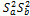; by Aroian, (1947) popularized by Baron & Kenny ; one that subtracts the third variable by Goodman , and one that does not include the third variable at all generally referred to as Sobel test . Aroian version of the Sobel test was recommended in Baron and Kenny  because it does not make the unnecessary assumption that the product of Sa and Sb is vanishingly small. The Goodman version of the test subtracts the third term for an unbiased estimate of the variance of the mediated effect, but according to Preacher & Leonardelli , this can sometimes have the unfortunate effect of yielding a negative variance estimate. This study therefore compares the three tests of indirect effect via the three variations in the standard error computations in other to determine the best.

The purpose of this study includes:

1. To obtain the coefficients of single mediation using the product of their unstandardised regression coefficients,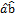and the difference of the regression coefficients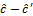and compare the results.

2. To conduct the Sobel’s test, Aroian test and Goodman test of indirect effect for a single mediator and compare their results.

2. Literature Review

The interest of mediation is in calculation the size of the mediating effect also known as indirect effect. The indirect effect measures the extent to which the dependent variable changes when the independent variable is held fixed; and the mediator variable changes by the amount it would have changed had the independent variable increased by one unit .

The indirect effect measures the extent to which the dependent variable changes when the independent variable is held fixed; and the mediator variable changes by the amount it would have changed had the independent variable increased by one unit  (Judd & Kenny, 1981)

According to MacKinnon & Dwyer , the indirect effect in the single-mediator model may be calculated in two ways, as either âb̂ or ĉ − ĉ′. The value of the mediated or indirect effect estimated by taking the difference in the coefficients, ĉ − ĉ′, corresponds to the reduction in the independent variable effect on the dependent variable when adjusted for the mediator. The difference between the coefficients obtained from the two different regression coefficients (ĉ − ĉ′) should be equal to the product of the coefficients ab. To test for significance of indirect effect, the difference is then divided by the standard error of the difference and the ratio is compared to a standard normal distribution.

Researchers often test whether there is complete or partial mediation by testing whether the c′ coefficient is statistically significant, which is a test of whether the association between the independent and dependent variable is completely accounted for by the mediator. If the c′ coefficient is statistically significant and there is significant mediation, then there is evidence for partial mediation. It is often unrealistic to expect that a single mediator would be explained completely by an independent variable to dependent variable relation .

From the Baron & Kenny  approach, mediation is supported if the partial direct effect for path c is non significantly different from zero and path b is significantly greater than zero. If c is not significantly different from zero, results are consistent with a full mediational model. If path b is significant after controlling for the direct effect of X (path c), but path c is still significant, the model is consistent with partial mediation.

Many studies investigating mediation use a randomized experimental design, where participants are randomized to levels of one or more factors in order to demonstrate a pattern of results consistent with one theory and inconsistent with another theory Differences in means between groups are then attributed to the experimental manipulation of the mediator. The results of the randomized study along with the predictions of different theories are used to provide evidence for a mediation hypothesis and suggest further studies to localize and validate the mediating process .

Sherman & Gorkin  randomly assigned subjects to solve either (a) a sex- role related brainteaser, or (b) a brainteaser not related to sex roles. The sexist brainteaser condition was designed to evoke cognitive dissonance in the self-identified feminist subjects, while the non sex-role related condition was not. Participants were then asked to judge the fairness of a legal decision made in an affirmative action trial. The results were consistent with the prediction that participants with strong feminist beliefs were more likely to make extreme feminist judgments in the trial if they failed the sexist brainteaser task, in an attempt to reduce cognitive dissonance. Although results of this experiment were taken as evidence of a cognitive dissonance mediation relation, the mediating variable of cognitive conflict was not measured to obtain more information on the link between the manipulation, cognitive dissonance, and feminist judgments.

Researchers are often interested in mediation analysis to understand how a treatment works, in particular how much of a treatment’s effect is mediated by an intermediated variable and how much the treatment directly affects the outcome not through the mediator. The standard regression approach to mediation analysis assumes sequential ignorable nature of the mediator, which is that the mediator is effectively randomly assigned given baseline covariates and the randomized treatment. The author argued that since the experiment does not randomize the mediator .

MacKinnon et al.  reviewed and compared 14 methods to test the mediation effects through a Monte Carlo study and found that testing H0: ab = 0 was the best way to evaluate the mediation effects.

MacKinnon, Lockwood and Williams  also compared the bootstrap resampling method with the single sample method and found that the bootstrap method obtained more accurate confidence limits. They further suggested that confidence limits of the mediation effects provided much more information than the estimates themselves.

3. Research Methods

The study employs mediation analysis. The analysis begins by testing for multicolinearity among the independent variables. This was accomplished with the use of variance Inflation Factor (VIFk). The first step in meditational analysis is to establish that a relationship exist the independent variable and the dependent variable using a regression linear model. This is known as path c. Next is to conduct a regression analysis with the suspected variables predicting the dependent variable. This is known as path b. thirdly, conduct a regression analysis with the independent and other variables predicting the dependent variable, known as partial effect model.

3.1. Test of Significance of the Indirect Effect

A test of significance of the indirect effect can be constructed using a ratio of the indirect coefficient to its standard error. The tests of indirect effect is given as: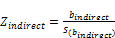(4)

3.2. Three Approaches to Standard Error Computation

i. Sobel’s test: the standard error for the Sobel test is given as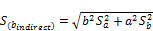(5)

ii. Aroian test;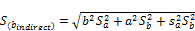(6)

iii. Goodman test: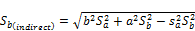(7)

Where: b is the unstandardized coefficient for path b and a is the unstandardized coefficient for path a.

4. Result and Discussion of Findings

The SPSS software was used to compute the VIFk. The first colinearity test conducted showed that Z2 has a VIFk greater than 10. Hence it was deleted and the VIF recalculated. The second VIFk result is presented below.

Table 1. Colinearity statisticsa.

 Model Collinearity Statistics Tolerance VIF Z1 .224 4.460 Z3 .507 1.972 Z4 .208 4.812 Z5 .395 2.530 Z6 .667 1.500 Z7 .204 4.895

a. Dependent Variable: Y

From table 1 it is observed that removal of Domestic Product (Z2) alone further reduced the VIF values of the other variables to values below 10 which is an acceptable VIF value

4.1. Analysis of Path c

The regression model of path c is given as:

Y= 5.11 + 6.13x10-13X

The significance of the variables are accessed by comparing the level of significance (α=0.05) with the p-value. Comparing the p-value (0.00) with the level of significance shows that significant relationships exist between the dependent (Y) and independent variable (X).

4.2. Analysis of Path a

There are six variables suspected to mediate between dependent and independent variables, hence six models of ‘path a’ are required of which the interest are in the coefficients of X, (a). The coefficients of paths ‘a’ are given below.

Here again, we ascertain the significance of X when predicting the suspected mediator variables by comparing the p-values [PV (a)] with the significance level. In the analysis of path a, the independent variable was found not to significantly predict Z5, hence suggesting that Z5 cannot be mediator variable.

Table 2. Coefficients of Path a.

 Z=a0+a1X(path a) a1 Sa PV(a) Z1 2.118E-12 0.000 0.000 Z3 1.865E-14 0.000 0.101 Z4 2.68E-11 0.000 0.000 Z5 2.571E-14 0.000 0.767 Z6 -5.482E-13 0.000 0.045 Z7 2.064E-12 0.000 0.000

4.3. Analysis of Path b

Similarly, we ascertain the significance of the suspected mediator variables (Zi: i=1, 2,…, 7) when predicting the dependent variable (Y) by comparing the p-values [PV (b)] with the significance level (α=0.05), see table 3 below.

It was also observed that only Z5 does not significantly predict the dependent variable as the p-value is greater than the level of significance. The non -significance of Z5 further affirms that Z5 cannot be a mediator, hence it is deleted.

Table 3. Coefficients of Z in path b.

 Y=b0+b1Z(path b) b1 Sb PV(b) Z1 0.211 0.034 0.000 Z3 4.214 1.677 0.017 Z4 0.012 0.002 0.000 Z5 0.082 0.245 0.740 Z6 -0.183 0.065 0.008 Z7 0.243 0.032 0.000

4.4. Partial Effect Model

The table below presents the coefficients of the independent variable (X) and the ith mediator variables when predicting the dependent variable (Y). The coefficients of X and Z are seen in the table 4.

From table 4 it is observed that the coefficients of X in the partial effect model for the various suspected mediators (Z1, Z3, Z4, Z6 and Z7) are all less that the coefficient of X in path C (i.e C′ < C). However only the coefficients of Z3 and Z6 are significant in the partial effect model at 5% level of significance, hence they are confirmed as mediators.

Table 4. Coefficients of X and Z in the partial effect model.

 Y=a+C'X+bZ (partial effect) C C' std err PV(C') b std err PV(b) Z1 6.13x10-13 8 x10-13 0.00 0.00 -0.088 0.044 0.056 Z3 6.13x10-13 5.83 x10-13 0.00 0.00 1.654 0.765 0.038 Z4 6.13x10-13 5.98 x10-13 0.00 0.00 0.001 0.002 0.779 Z6 6.13x10-13 5.77 x10-13 0.00 0.00 -0.066 0.031 0.039 Z7 6.13x10-13 7.7 x10-13 0.00 0.00 -0.076 0.059 0.208

Table 5. Indirect Effects.

 Z a b C C' C-C' ab Z3 1.87x10-14 1.654 6.134x10-13 5.825x10-13 3.09x10-14 3.085x10-14 Z6 -5.48x10-13 -0.066 6.134x10-13 5.771x10-13 3.63 x10-14 3.618x10-14

Table 6. Standard Errors of coefficients of mediators.

 M C C' ai bi Sa Sb Sa2 Sb2 a12Sb2 b12Sa2 Sa2Sb2 Z3 6.13x10-13 5.825 x10-13 1.87 x10-14 4.42 x10-13 0.00 1.677 0.000 2.812 9.78 x10-28 0 0 Z6 6.13 x10-13 5.771 x10-13 5.48 x10-13 -0.066 0.00 0.065 0.000 0.0042 1.27 x10-27 0 0

4.5. Indirect Effect

Two methods are employed in the calculation of effect size: difference of coefficient, C-C′ and the product of coefficient which is the product of coefficient of X in equation (2) and the coefficient of Z in equation (3).

A comparison of the two methods in table 5 shows that both methods give approximate values of the effect sizes. The difference of coefficient method gives a slightly higher effect size value of about 0.02 points.

After the effect sizes have been calculated, next is to test the significance of the effect sizes of the variables that show evidence of mediation. Thus the significance of the effect size is tested for Z3 and Z6.

Standard Errors of effect sizes

Three methods of standard error computation as shown in are given. The various coefficients and standard errors have been extracted from the respective regression analyses in equations (5), (6) and (7).

From equation (5) the standard error for the effect size of Z3 is given as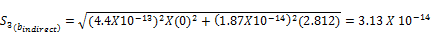Where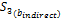is the standard error of the indirect effect of Z3.

Similarly the standard error for the effect size of Z6 is given as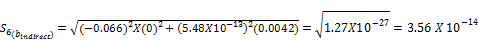From (6) the Aroian test is calculated as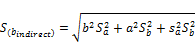Hence Z3 we have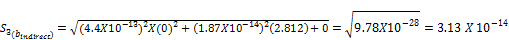For Z6 we have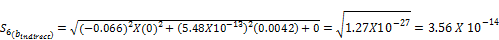From (7) the Goodmans method of standard error of effect size is calculated as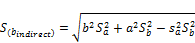The standard error for Z3 population is given as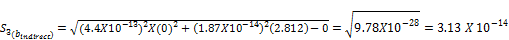For Z6 we have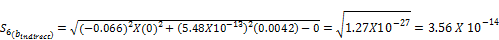4.6. Test of Significance of Indirect Effect

The tests of indirect effect for the various mediators are presented below

Sobel’s test

From equation (4), the calculation of test of indirect effect is presented:

For Z3 and Z6 we have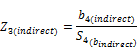case 1: C-C′ Case 2: ab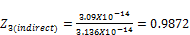;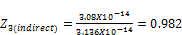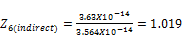;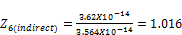Aroian’s test and Goodman’s test:

Since the standard errors for Sobel, Aroian and Goodman’s test are the same, it implies same results for the three tests of indirect effect.

The table below gives the summary result for the various tests of indirect effects using the difference of coefficient method.

Table 7. Tests of Indirect Effects (C-C’).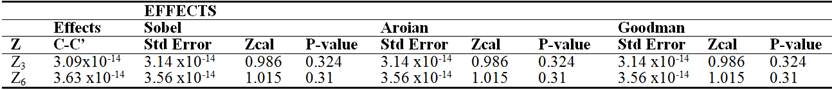5. Summary of Findings

This study compares various methods of mediations analysis. Firstly, it compares the two methods of calculating indirect effect which are product of coefficient and difference of coefficients respectively. Secondly, the study compares the three methods of testing the significance of indirect effect vis avis Sobel’s test, aroian test and Goodman’s test. The differences in these three tests are due to variations in the methods of standard error computation. The findings are discussed.

Although not statistically significant, Z3 and Z6 were respectively found to have trace of partial mediation as C′ was less than C in the single mediator case.

The comparison of two methods of calculating indirect effect show that both methods of product of coefficients and difference of coefficients give approximately the same result. However the product of coefficient gave a slightly higher result.

The comparison of test of indirect effect for mediator shows that the tests gave the same result for Sobel’s, Aroian and Goodman test.

6. Conclusion

The results of the various comparisons of methods of mediational analysis conducted in this study has been presented. Hence the following conclusions: The comparison of the methods of computation of indirect effect has led to the conclusion that there is a slight difference in both, as the method of product of coefficient gives a slightly higher result than the method of difference of coefficient. The slight difference in the effect size was observed to be the cause of the slight differences in the result of the test of indirect effect, since the standard errors are the same for both single.

References

1. Fairchild, A. J and MacKinnon, D. P. (2009) A general model for testing mediation and moderation effects. Prevention Science, 10, 87-99.
2. MacKinnon, D. P. (2000). Contrasts in multiple mediator models. In J. Rose, L. Chassin, C. C. Presson, & S. J. Sherman (Eds.), Multivariate applications in substance use research: New methods for new questions (pp. 141-160). Mahwah, NJ: Erlbaum.
3. Judd CM, Kenny DA. (1981). Estimating the effects of social interventions. Cambridge University Press; Cambridge, England.
4. Baron, R. M., & Kenny, D. A. (1986). The moderator-mediator variable distinction in social psychological research: Conceptual, strategic and statistical considerations. Journal of Personality and Social Psychology, 51, 1173-1182.
5. Goodman, L. A. (1960). On the exact variance of products. Journal of the American Statistical Association, 55, 708-713.
6. Sobel, M. E. (1982). Asymptotic Confidence Intervals For Indirect Effects In Structural equations models. In S. Leinhart (Ed.), Sociological methodology 1982 (pp. 290-312). San Francisco: Jossey-Bass.
7. Preacher, C. J & Leonardelli, G. J (2010) Calculation for the Sobel test: An interactive calculation tool for Mediation tests, http://quantpsy.org/sobel/sobel.htm.
8. MacKinnon, D. P., & Dwyer, J. H. (1993). Estimating mediated effects in prevention studies. Evaluation Review, 17, 144-158.
9. James L.R & J. M Brett (1984). Mediators, moderators, and tests for mediation. J Appl Psychol. 1984;69:307–21.
10. MacKinnon, D. P., Fairchild, A. J., & Fritz, M. S. (2007). Mediation analysis. Annual Review of Psychology, 58, 593-614.
11. Sherman SJ, Gorkin L. (1980) Attitude bolstering when behavior is inconsistent with central attitudes. J Exp Soc Psychol. Vol.16:388–403.
12. James L.R & J. M Brett (1984). Mediators, moderators, and tests for mediation. J Appl Psychol. 1984;69:307–21.
13. Mackinnon, D. P., Lockwood, C. M., & Williams, J. (2004). Confidence Limits For the indirect effect: Distribution of the product and resampling methods. Multivariate Behavioral Research, 39, 99-128.

 Contents 1. 2. 3. 3.1. 3.2. 4. 4.1. 4.2. 4.3. 4.4. 4.5. 4.6. 5. 6.
Article ToolsAbstractPDF(226K)